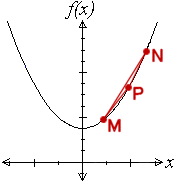Go to contentThe gradient at the point P can be approximated by using points that are near to P (such as M and N) and finding the gradient of the straight line between these points. The closer these points are to P the better the approximation will be.
We can find the gradient of PM and of PN. The gradient at P should lie between these two values. We can also find the gradient of MN which may give an even more accurate estimate.
This approach is called "differentiation from first principles"

This method may not be be part of your examination, but is here to give you a chance to see what lies behind the methods you use.

## Summary/BackgroundLeibniz (1646-1716) and Newton (1642-1727) independently discovered calculus. Their key idea was that differentiation and integration undo each other. Using this symbolic connection, they were able to solve an enormous number of important problems in mathematics, physics, and astronomy. While in Paris Leibniz developed the basic features of his version of the calculus. In 1673 he was still struggling to develop a good notation for his calculus and his first calculations were clumsy. On 21 November 1675 he wrote a manuscript using the \int f(x) dx notation for the first time. In the same manuscript the product rule for differentiation is given. By autumn 1676 Leibniz discovered the familiar d(x^n) = nx^{n-1} dx for both integral and fractional n.Newton made contributions to all branches of mathematics, but is especially famous for his solutions to the contemporary problems in analytical geometry of drawing tangents to curves (differentiation) and defining areas bounded by curves (integration). Not only did Newton discover that these problems were inverse to each other, but he discovered general methods of resolving problems of curvature, embraced in his "method of fluxions" and "inverse method of fluxions", respectively equivalent to Leibniz's later differential and integral calculus. Newton used the term "fluxion" (from Latin meaning "flow") because he imagined a quantity "flowing" from one magnitude to another.You can get a better display of the maths by downloading special TeX fonts from jsMath. In the meantime, we will do the best we can with the fonts you have, but it may not be pretty and some equations may not be rendered correctly.

## Glossary

### calculus

the study of change; a major branch of mathematics that includes the study of limits, derivatives, rates of change, gradient, integrals, area, summation, and infinite series. Historically, it has been referred to as "the calculus of infinitesimals", or "infinitesimal calculus".
There are widespread applications in science, economics, and engineering.

### curvature

how fast a curve is changing direction at a given point

### differentiation

The process of finding a derivative.

The slope of a line; the angle of its inclination to the horizontal.

### integral

the anti-derivative

### integration

the process of finding an integral, the reverse process to differentiation.

### magnitude

A measure of the size of a mathematical object

### newton

the unit of force

### product rule

The rule for differentiating products:
(uv)' = uv' + u'v

### rule

A method for connecting one value with another.

### solve

To find the answer or solution to a problem.

### straight

The type of line produced by a linear equation.

### union

The union of two sets A and B is the set containing all the elements of A and B.

Full Glossary List

## This question appears in the following syllabi:

SyllabusModuleSectionTopicExam Year
AP Calculus AB (USA)3DifferentiationFirst principles-
AP Calculus BC (USA)3DifferentiationFirst principles-
AQA A-Level (UK - Pre-2017)C1DifferentiationFirst principles-
AQA AS Maths 2017Pure MathsDifferentiationFrom First Principles-
AQA AS/A2 Maths 2017Pure MathsDifferentiationFrom First Principles-
CBSE XI (India)CalculusLimits and DerivativesDefinition of a derivative-
CCEA A-Level (NI)C1DifferentiationFirst principles-
CIE A-Level (UK)P1DifferentiationFirst principles-
Edexcel A-Level (UK - Pre-2017)C1DifferentiationFirst principles-
Edexcel AS Maths 2017Pure MathsDifferentiationFrom First Principles-
Edexcel AS/A2 Maths 2017Pure MathsDifferentiationFrom First Principles-
I.B. Higher Level6DifferentiationFirst principles-
I.B. Standard Level6DifferentiationFirst principles-
Methods (UK)M8DifferentiationFirst principles-
I.B. (MSSL)7DifferentiationFirst principles-
OCR A-Level (UK - Pre-2017)C1DifferentiationFirst principles-
OCR AS Maths 2017Pure MathsDifferentiation BasicsFrom First Principles-
OCR MEI AS Maths 2017Pure MathsDifferentiation BasicsFrom First Principles-
OCR-MEI A-Level (UK - Pre-2017)C2DifferentiationFirst principles-
Pre-U A-Level (UK)4DifferentiationFirst principles-